Sparse Spatial Autoregressions

By

R. Kelley Pace

LREC Endowed Chair of Real Estate

Louisiana State University

Baton Rouge, LA 70803-6308

(225)-388-6238

FAX: (225)-334-1227

Kelley@Pace.am

www.spatial-statistics.com

and

Ronald Barry

Department of Mathematical Sciences

(907)-474-7226

FAX: (907)-474-5394

Abstract:

Given local spatial error dependence, one can construct sparse spatial weight matrices. As an illustration of the power of such sparse structures, we computed a simultaneous autoregression involving observations on median housing prices by all California census blocks. We calculated the 20,640 observation autoregression in under 19 minutes despite needing to compute a 20,640 by 20,640 determinant 10 times.

Key Words: spatial autoregression, SAR, sparse matrices.

A version of this paper has appeared in:

Pace, R. Kelley, and Ronald Barry, "Sparse Spatial Autoregressions," Statistics and Probability Letters, Volume 33, Number 3, May 5 1997, p. 291-297.

Sparse Spatial Autoregressions

I. Introduction

Regression is perhaps the most often used technique in statistics. When applied to spatially distributed observations, however, much predictive power can be lost by ignoring the presence of spatial autocorrelation. Moreover, ignoring spatial autocorrelation leads to a serious violation of the assumptions underlying ordinary least squares regression which can result in erroneous statistical inference. Fortunately, a variety of spatial estimators can adjust for this problem. Examples include simultaneous autoregressions (SAR), conditional autoregressions (CAR), and kriging (see Cressie (1993)).

Unfortunately, all of these techniques involve examining the explicit relation between an observation and all other observations. If n observations exist, this leads to n2 potential relations. Hence, as n becomes large, computing spatial estimators can become quite expensive as these usually require computing the determinant or inverse of an n by n matrix which requires order of n3 operations (O(n3)). This conflicts with the increasing prevalence of large data sets involving thousands of observations. Clearly, standard spatial statistical methods can become impractical for many realistic applications.

Fortunately, spatial autocorrelation usually declines with distance. Truncating the influence of observations past a certain distance could greatly reduce the number of relations (non-zero elements) needed to estimate the spatial regression. Mathematically, sparse matrices can represent this situation. Sparse matrix techniques store only the non-zero elements of a matrix and also avoid performing unnecessary computations on the zero elements. This both reduces storage space and accelerates execution time.

To illustrate the dramatic improvements possible, we computed a simultaneous autoregression (SAR) using 20,640 observations. Each SAR likelihood function evaluation requires computing the determinant of the 20,640 by 20,640 matrix. The sparsity of the problem (.019% non-zero elements) makes it possible to compute ten evaluations of the likelihood in under 19 minutes. Moreover, a dense solution to the problem would have required storing around 3.4 gigabytes of data while the sparse solution to the problem used just over one megabyte of memory.

To place these results into perspective, Li (1995) used a IBM RS6000 Model 550 and a CM5 parallel processing supercomputer to compute a 2500 observation SAR. The CM5 had 32 processors each with 32MB of local memory and four vector units. For a 2500 by 2500 spatial weight matrix the RS6000 required 8515.07 seconds while the CM5 required 48 seconds. Since computing determinants requires O(n3) operations, the differences in size would require a factor of (20640/2500)3 more operations, assuming no additional bottlenecks. Given this size adjustment, the extrapolated computation times would go to over 55 days for the RS6000 and 7.5 hours for the CM5 on the 20,640 by 20,640 problem. Hence, personal computers with sparse technology can exceed supercomputer performance for dense technology.

The tremendous gains in computing speed do not come at the expense of statistical performance. For the simple model of California housing prices examined, the SAR manages to achieve a median absolute error of 0.1084 while OLS produced a median absolute error of 0.2101, almost twice as great.

Section II discusses the spatial autoregressive error estimator employed, section III provides details on an improved algorithm for computing spatial estimators, section IV estimates the resulting spatial error autoregression, while section V concludes with the key results.

II. A Spatial Autoregressive Error Estimator

Section II.A describes the likelihood function for a spatial autoregressive error process. This likelihood function depends critically upon D, the spatial weighting matrix. Hence, section II.B describes D in more detail.

A. The Spatial Autoregressive Error Likelihood Function

When errors exhibit spatial autocorrelation, the simultaneous autoregression (SAR) estimator corrects the usual prediction of the dependent variable,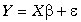, by a weighted average of the "deviations"on nearby observations as in ,()1

where D represents an n by n weighting matrix with 0s on the diagonal (the observation cannot predict itself) and non-negative off-diagonals. To maintain the interpretation of a weighted average, the rows of D sum to 1 as implied by below. Such weighting matrices are said to be row-standardized (Haining (1990, p. 82)). A non-zero entry in the jth column of the ith row indicates that the jth observation will be used to adjust the prediction of the ith observation (i ¹ j). After correcting for these interactions, the SAR models assume the residuals, e , are independently and normally distributed. These assumptions appear in .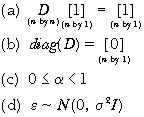()2

The simultaneous autoregression (SAR) has the following log-likelihood function,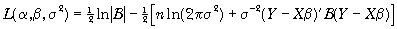()3

where B equals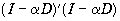. To ensure the sum-of-squared errors,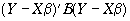, is strictly positive, B must be positive definite. Given the definition of D, for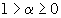,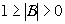, and hence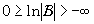. The maximum likelihood method efficiently estimates the model asymptotically (given the assumptions hold).

Assuming the existence of the ML estimate, one could predict Y via .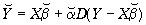()4

Furthermore, leads to the estimated errors in .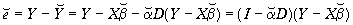()5

B. Specification of the Spatial Weight Matrix

The weight given to the census block groups for differencing depends upon their proximity as measured by the latitude and longitude for each observation relative to all other observations. Let dij represent the Euclidean distance between every pair of observations i and j and let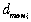represent the distance between the ith observation and its mth nearest neighbor. Moreover, let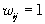ifand zero otherwise as stated by .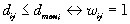()6

Naturally, this yields a weight of 1 for the census block group itself (dii=0) and 0 for each observation j more than dmaxi distance from observation i. Subsequently, in (7) we normalized the initial weights so thatthus making it into a standardized weight matrix.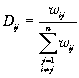(7)

In addition, we set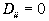, as assumed in , to prevent each observation from predicting itself.

For the third observation, D might appear as,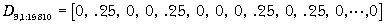.

Note, the third entry of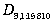equals 0 while the row sums to 1.

III. Computation of Spatially Autocorrelated Error Regressions

Examination of shows the main barrier to speedy computation of the estimates lies in the n by n nature of the spatial weighting matrix, D. In particular, computing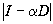, a determinant of an n by n matrix, requires substantial time for large n. Standard determinant computations use O(n3) operations while multiplication of a n by n matrix and a n by p matrix uses O(n2p). Thus, the storage requirements, temporary or permanent, rise with the square of n while the operation count rises with the cube of n. A problem with many observations would quickly exhaust storage space or require impractical amounts of computing time.

Fortunately, one can avoid such Herculean computational tasks. The spatial weighting matrix D contains mainly 0s and hence is sparse. The spatial weight matrix described in II.B uses the m nearest neighbors out of a possible n neighbors for each of the n observations. Thus, mn non-zero entries exist out of a possible n2 elements. A common measure of matrix sparsity examines the number of non-zero elements of a matrix relative to the total number of elements. Hence, the m nearest neighbor weighting matrix D has proportionally m/n non-zero elements (i.e., 4/20640). D is quite sparse for this problem and becomes progressively more sparse for large empirical applications.

This level of sparseness totally alters the number of operation counts needed for the computation of determinants and matrix multiplication. The operation count depends more upon the number of non-zero elements than the total number of elements. Moreover, the sparse matrix procedures store only the non-zero elements and some form of index. Hence, sparse matrix methods can make it possible to handle very large applications.

Sparse methods fall into two basic categories, direct and iterative. Statisticians commonly use iterative methods such as the conjugate gradient in the optimization of non-linear problems. However, the conjugate gradient, as well as a host of other techniques such as the Jacobi, successive overrelaxation, Krylov subspace methods, and so forth, also have applications in linear systems. A very sparse matrix makes it extremely fast to compute each iteration. Like any minimization (maximization) problem, the algorithms need positive (negative) definite matrices to ensure a global solution. Moreover, the more pronounced the positive (negative) definiteness, the faster convergence usually proceeds. See Saad (1996) for more details.

Direct methods take advantage of blocks of zeros in the gaussian elimination process. Hence, direct methods usually prefer contiguous groups of zeros in the matrix. Specific patterns such as bandedness and bordered matrices have dedicated methods for their solution (Press (1988, p. 72-74)). More general patterns require the use of factorization methods such as the reverse Cuthill-McKee, minimum degree (followed here), and nested dissection, to reorder the matrix for fast gaussian elimination (George and Liu (1981)).

Given the close connection of the determinant with gaussian elimination, computing spatial autoregressions seems more suited to direct methods. A potential problem arises, however, as the goal of reordering the matrix to maximize computation speed could conflict with the ordering (pivoting) of the matrix to maximize numerical stability. Fortunately, the structure of the problem leads to either diagonal dominance or symmetry. Both situations have very favorable error properties and do not require pivoting. Examination of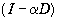shows for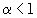the sum of the off-diagonal elements in each row is less than the diagonal (1). Hence, for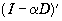the sum of the off-diagonal elements in each column is less than the diagonal (1) andis diagonal dominant. Since, computing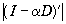sidesteps the error issues which arise from reordering (Golub and Van Loan (1989, p. 119-120)). Also, we could compute. The symmetry and positive definiteness of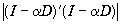allows the use of the Cholesky decomposition which also does not need pivoting to achieve numerical stability (George and Liu (1981, p. 9)).

We used the MATLAB programming language running on a 133Mhz Pentium computer to generate the estimates. The sparse matrix formulation required only 1130 seconds to evaluate 10 iterations of the likelihood function. Each iteration involved computing the determinant of a 20,640 by 20,640 matrix as well as various multiplications. In addition, the storage of the matrix took somewhat over 1 megabyte whereas the full matrix would have required 3.4 gigabytes of memory.

These speed increases coupled with decreases in storage requirements make the estimation of large spatial problems practical. For medium and smaller problems it allows users to jointly model other phenomenon of interest such as specification or simultaneity.

IV. Maximum Likelihood Sample Estimation of a Spatial Autoregression

This section illustrates the spatial autoregression estimator from section II using the 1990 census data. Section A discusses the model, section B presents the data, and section C presents the actual estimation results.

A. Model

We fitted the following model:

ln(median value)= intercept + b 2median income+ b 3median income2 + b 4median income3 + b 5ln(median(age)) + b 6ln(total rooms/population) + b 7ln(bedrooms/population) + b 8ln(population/households) b 9ln(households) (8)

B. Data

We collected information on the variables in (8) using all the block groups in California from the 1990 Census. In this sample a block group on average includes 1425.5 individuals living in a geographically compact area. Naturally, the geographical area included varies inversely with the population density. We computed distances among the centroids of each block group as measured in latitude and longitude. We excluded all the block groups reporting zero entries for the independent and dependent variables. The final data contained 20,640 observations on 9 characteristics.

C. Maximum Likelihood Sample Estimation

Table 1 contains the sample estimates from using OLS and the SAR maximum likelihood estimators. To emphasize the sparsity of the problem a priori we picked four nearest neighbors to receive positive weights in the spatial weight matrix D. Based upon a numerical search, the SAR maximum likelihood estimate of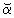was .8536.

Note the treatment by the two estimators of the age variable. OLS produces a positive and significant estimate of the age variable with a t statistic of 33.6133 while the maximum likelihood SAR produces a negative, significant estimate with a t statistic of -11.0942.

Even when both estimators agree in terms of the direction of an effect, they may differ in their implications concerning the functional form governing the effect. For example, OLS arrives at a linear estimate of the effects of median income upon ln(price) with negative quadratic and cubic effects. In contrast, the SAR estimate shows a positive linear and quadratic effect of median income upon ln(price) with a negative cubic effect.

For many applications prediction error constitutes the main concern. In this respect the SAR estimator greatly outperforms OLS. For example, the OLS sample R2 was .6078 while for the SAR estimate the sample R2 was .8536, a dramatically better goodness-of-fit. Equally dramatic, the median absolute errors under OLS of .2101 fall by 48.4% to .1084 under the SAR estimator.

For other applications statistical inference constitutes the main concern. The presence of such pervasive spatial autocorrelation completely invalidates the use of the usual iid based OLS p-values. Indeed, the dramatic change in many of the t-values between the two estimators emphasizes how conditional these are upon the assumed correlation structure.

V. Conclusion

Accurate prediction and correct inference in a spatial setting must use the information contained in the errors on nearby observations. The canonical estimators available, however, require order of n3 computations. Given many applications must deal with thousands of observations, this presents a substantial barrier to the adoption of the standard spatial techniques. However, as a stylized fact, only the relations among nearby observations matter greatly which means one can employ sparse matrix techniques to achieve a great savings of both storage space and execution time.

As an illustration of the power of these techniques, the 20,640 observations employed herein would have required the use of a matrix taking 3.4 gigabytes using normal dense methods. The sparse matrix formulation of the problem dropped the storage space to just above 1 megabyte. Finding the spatial maximum likelihood estimate required 10 likelihood evaluations each needing the computation of the determinant of a 20,640 by 20,640 matrix. This took under 19 minutes on a 133 megahertz Pentium computer.

As expected, incorporating the spatial information greatly reduced prediction errors. For the simple model employed, the spatial estimator displayed a median absolute error almost one-half less than OLS. Moreover, the dramatic shift in t-values between the two estimators emphasized how conditional these are upon the assumed correlation structure.

The great gains produced by sparsity pose the question of what statistical factors lead to sparsity. First, if the regression function performs perfectly, it will leave only white noise and I would be the optimal spatial weight matrix. At the other extreme, a poor regression function might produce slowly decaying correlated errors and greatly reduce the sparsity of the spatial weight matrix. Often autocorrelated errors arise because of omitted variables. For example, pollution from a factory might decline with the inverse of the squared distance. Applying a model without a pollution independent variable might result in correlated errors decaying with the inverse of the squared distance. Second, the span and density of the data influence sparsity. An optimal spatial weighting matrix might include more neighboring observations in predicting Hong Kong apartment rents than in predicting suburban Houston apartment rents. On a national scale the neighboring observations for any given apartment would occupy a small relative bandwidth relative to the equivalent problem over one census tract. Sparsity of various forms arise in many physical and electronic systems (Rice (1981, p. 25-28)).

The advent of geographical information systems which provide data sets of ever-increasing size, the superior prediction as well as inference from spatial estimators using this information, and the low-cost computation of spatial estimators using sparse matrices have the potential to greatly increase the appeal of spatial estimators.

Acknowledgments

We would like to gratefully acknowledge the research support we have received from the University of Alaska. We would also like to thank Otis Gilley, an anonymous referee, and the editor for their thoughtful comments as well as the seminar participants at the University of Connecticut at Storrs and Southern Illinois University at Edwardsville.

Bibliography

Cressie, Noel A.C. (1993) Statistics for Spatial Data (Wiley, New York, 2nd ed.).

Gilley, O.W., and R. Kelley Pace (1995), "Improving Hedonic Estimation with an Inequality Restricted Estimator," Review of Economics and Statistics 77, 609-621.

George, Alan, and Joseph Liu (1981), Computer Solution of Large Sparse Positive Definite Systems (Prentice-Hall, Englewood Cliffs).

Golub, G. H., and C. F. Van Loan (1989) Matrix Computations (John Hopkins, Balitimore, 2nd ed.).

Haining, Robert (1990) Spatial Data Analysis in the Social and Environmental Sciences (Cambridge, Cambridge University Press).

Li, Bin, (1995) "Implementing Spatial Statistics on Parallel Computers," in: Arlinghaus, S., ed. Practical Handbook of Spatial Statistics (CRC Press, Boca Raton), pp. 107-148.

Pace, R. Kelley, and O.W. Gilley (forthcoming) "Using the Spatial Configuration of the Data to Improve Estimation," Journal of the Real Estate Finance and Economics.

Pace, R. Kelley, and O.W. Gilley (1993) "Improving Prediction and Assessing Specification Quality in Non-Linear Statistical Valuation Models," Journal of Business and Economics Statistics 11, 301-310.

Press et al. (1988), Numerical Recipes in C, (Cambridge University Press, Cambridge).

Rice, John (1981), Matrix Computations and Mathematical Software, (McGraw-Hill, New York).

Saad, Yousef (1996), Iterative Methods for Sparse Linear Systems, (PWS Publishing, Boston).

 Table 1 — OLS and SAR Estimates for Median Housing Prices Across 20,640 California Census Block Groups Bols tols Bsar tsar intercept 11.4939 275.7518 11.6637 402.5925 Median Income 0.4790 45.7768 0.0349 4.7104 Median Income2 -0.0166 -9.4841 0.0100 8.4280 Median Income3 -0.0002 -1.9157 -0.0007 -12.2444 ln(Median Age) 0.1570 33.6123 -0.0421 -11.0942 ln(Total Rooms/ Population) -0.8582 -56.1280 0.3098 24.5768 ln(Bedrooms/ Population) 0.8043 38.0685 -0.1926 -11.8049 ln(Population/ Households) -0.4077 -20.8762 -0.0342 -2.3582 ln(Households) 0.0477 13.0792 0.0034 1.5569 a 0.8536 R2 0.6078 0.8594 Median |e| 0.2101 0.1084 Execution Time 1130 seconds Number of Likelihood Evaluations 10

../../../../index.htm

../../../../manuscript_index.htm